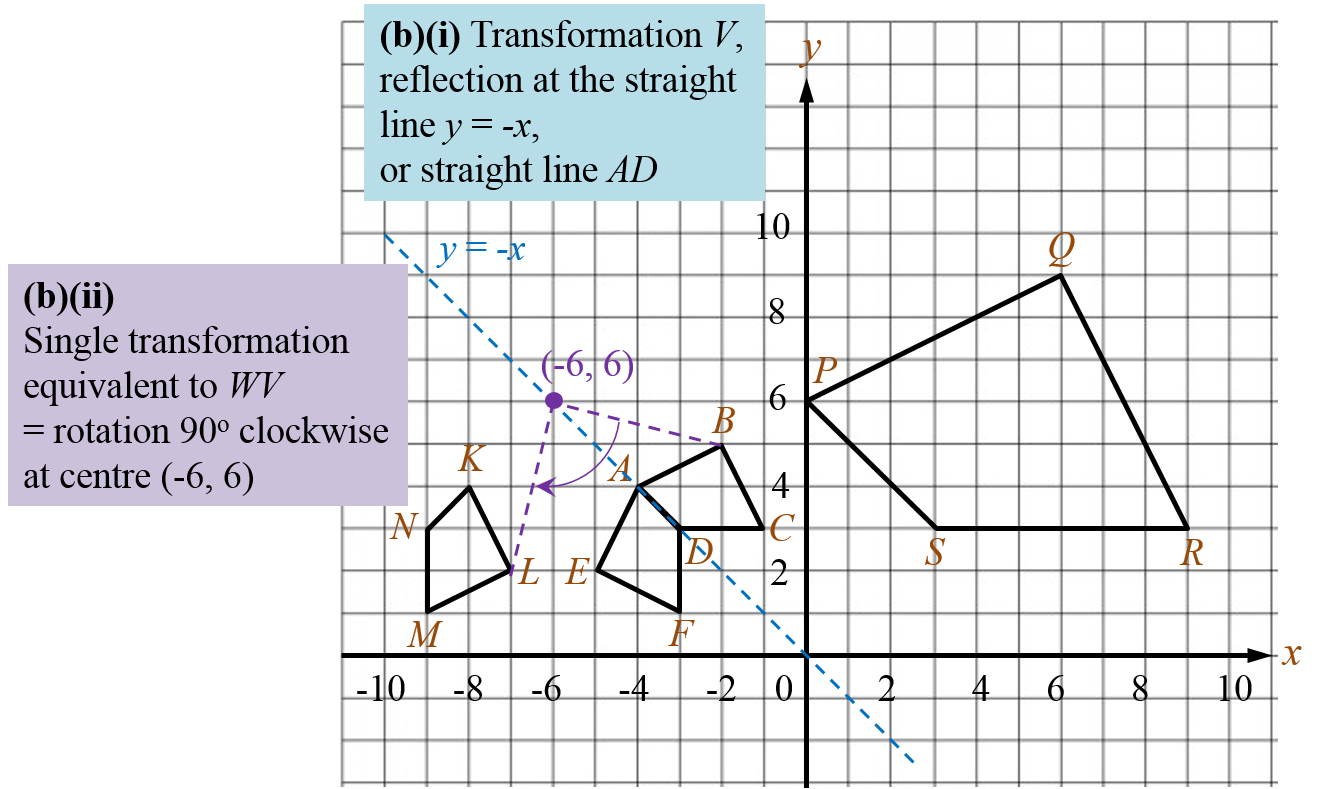# SPM Mathematics Trial 2021 (Selangor), Paper 2 (Question 15)

Question 15:
Diagram 8 shows quadrilaterals ABCD, AEFD, KLMN and PQRS drawn on a Cartesian plane.Diagram 8

(a) Transformation G is a reflection in the straight line x = 0.
Transformation H is a translation  $\left(\begin{array}{l}-3\\ 2\end{array}\right)$ .
State the coordinates of the image of point F under the following transformations:
(i) H
(ii) HG
[3 marks]

(b) AEFD is the image of ABCD under transformation V.
KLMN is the image of AEFD under transformation W.
Describe in full,
(i) the transformation V,
(ii) a single transformation which is equivalent to the combined transformation WV.
[5 marks]

(c) PQRS is the image ABCD under an enlargement.
(i) State the scale factor and the coordinates of the centre of the enlargement.
(ii) Given that PQRS represents a region of an area 412.2 m2, calculate the area, in m2, of the region represented by ABCD.
[4 marks]

Solution:
(a)Thus, the coordinates of the image of point F under the following transformations:
(i) H → (-6, 3)
(ii) HG → (3, 1) → (0, 3)

(b)(c)(i)

(c)(ii)